Opinion Dynamics: the Effect of the Number of Peers Met at Once

Journal of Artificial Societies and Social Simulation vol. 11, no. 2 4
<https://www.jasss.org/11/2/4.html>

Received: 29-May-2007    Accepted: 23-Dec-2007    Published: 31-Mar-2008###Abstract

The opinion dynamics model introduced by Deffuant and Weisbuch as well as the one by Hegselmann and Krause are rather similar. In both models individuals are assumed to have opinions about an issue, they meet and discuss, and they may adapt their opinions towards the other agents` opinions or may ignore each other if their positions are too different. Both models differ with respect to the number of peers they meet at once. Furthermore the model by Deffuant and Weisbuch has a convergence parameter that controls how fast agents adapt their opinions. By defining the reversed parameter as self-support we can extend the applicability of this parameter to scenarios with more than one interaction partner. We investigate the effects of changing the number of peers met at once, which is done for different population sizes, and the effects of changing the self-support. For describing the dynamics we look at different statistics, i.e. number of cluster, number of major clusters, and Gini coefficient.

Keywords:
Opinion Dynamics, Communication Regime

###Introduction

1.1
Opinion dynamic (OD) models describe the process of opinion formation in groups of individuals. We focus on continuous opinion dynamics with compromising agents in a time-discrete world. We assume that an opinion is a continuous value between zero and one. In every time step, each agent adapts his opinion toward the opinions of a set of randomly selected other agents, while the new opinion is between the minimum and maximum of the own and all perceived opinions (compromising or averaging). A common feature among many models of continuous opinion dynamics is bounded confidence, which describes the fact that peers holding opinions that are sufficiently different from an agent's own opinion do not exhibit any influence on this agent. This idea reflects psychological concepts such as selective exposure. For a detailed discussion of how models of the type we look at relate to social psychological theory see the recent review by Mason et al. (2007).

1.2
Two models of continuous opinion dynamics have received significant attention, the model introduced by Deffuant and Weisbuch (DW model) (Deffuant et al., 2001, Weisbuch et al., 2001, Deffuant et al., 2002, Weisbuch et al., 2002) and the model introduced by Hegselmann and Krause (HK model) (Hegselmann and Krause, 2002). A fundamental difference between these two models is the number of agents that communicate. In each time step, in the DW model two randomly chosen agents mutually perceive their opinions, while in the HK model all agents perceive all other agents' opinions. The same tendency toward extreme models regarding the number of communicating agents can be found in the related literature on discrete opinion dynamics (see for instance Schweitzer and Holyst, 2000 but also Fortunato, 2004).

1.3
The difference between the DW model and the HK model is in a dimension that we would label the communication regime. Social reality may restrict the process of communication between agents such that communication between all agents at the same time (as done in the HK model) but also communication between only two randomly chosen agents out of all possible agents (as done by the DW model) appear as extreme cases.

1.4
In this article we want to investigate these rules about who is communicating with whom at what time. We call such rules and restrictions the communication regime of an opinion dynamics model. We call it 'regime' because it is independent of the agents' opinions; it is a parameter of the model. The communication regime includes the underlying social network but also how this network may change over time. In a mathematical sense the communication regime is a (temporal) sequence of networks of who perceives whose opinion. It can be treated as a module of the opinion dynamic models.

1.5
Another module of opinion dynamic models is the updating mechanism. The updating mechanism introduces rules how agents adapt their opinions based on a set of other agents' opinions. It models perceptual or information processing biases, e.g. bounded confidence or selective exposure. By many parallel and sequential communications these biases are multiplied in a group such that new and sometimes even more complex group dynamics can be observed.

1.6
More and more, the updating mechanism is treated as a module of OD models independently of the communication regimes. For instance, the updating mechanism of the basic DW model is put on network structures (see Amblard and Deffuant, 2004; Stauffer et al., 2004), and the HK model is developed with an updating mechanism that captures discrete opinions instead of continuous opinions (see Fortunato, 2004).

1.7
Observing the different extensions of the HK model and the DW model, we present a model that unifies these two models. As this requires an additional parameter, the number of peers met at once, we also investigate the effect that this parameter has on the dynamics.

1.8
In section 2 we introduce a model that contains the DW model as well as the HK model as special cases. As a prerequisite we unify the updating mechanism; thereby we redefine the convergence parameter μ, (now called self-support) of the DW model and introduce this idea into the HK model. Section 4 summarizes simulations that show how the difference in the communication regime, i.e. the number of individuals communicating at once, influences the dynamics of the model. Thereby we compare the dynamics for smaller and larger agent populations. We also show that the impact of self-support is moderated by the communication regime. In order to describe the aggregate dynamics we briefly discuss different aggregated measures (maximum cluster size, number of clusters, number of large clusters, Herfindahl index, and Gini coefficient) and show how they differ with respect to our simulations results.

###Generalizing the DW and HK model

2.1
As motivated in the introduction, the DW model and the HK model are very much alike, but differ mainly in the communication regime that determines who communicates with whom. In this section we will introduce a model that comprises both mentioned models as special cases. To find a parameterised model covering both, the DW model (with globally uniform uncertainty and without relative agreement) and the HK model, we build on the distinction between updating mechanism, which describes how individuals react if they interact with others, and the communication, which describes who is interacting with whom at what time.

2.2
However, before discussing the different modules of opinion dynamics, we briefly define what this is all about. Consider a set n: ={1,...,n} of agents. We call the vector X(t) ∈ Rn an opinion profile. Xi(t) for tN0 represents the opinion of agent i at time t. The initial opinion profile is given by X(0). We assume that the initial opinions are equally distributed in the opinion space, i.e. Xi(0) ∈ [0,1].
2.3
A communication regime describes who communicates with whom at what time, or - more general - who gets the information about the opinion of whom at what time. In the most general case this can be represented for a specific time step by a network or its adjacency matrix. The communication regime in its most general sense is then a sequence of networks or respectively their adjacency matrices.

2.4
For the HK model the communication regime is a sequence of fully connected networks, so that the adjacency matrices are completely filled with ones. In the DW model in every step two randomly chosen agents interact. Hence, every adjacency matrix is a unit matrix plus a one at one off-diagonal entry and a one at its corresponding entry on the other side of the diagonal. So, a group of two agents is a fully connected network, while all others do not interact.

2.5
In this paper we extend to communication regimes where in each time step m randomly chosen agents communicate (2 ≤mn, m = 2 is DW, m = n is HK). We call these communication regimes random m-communication regimes. So, the adjacency matrix of a network in a random m-communication regime is the unit matrix plus ones at all entries ij where i,j form a set of m random agents.

2.6
The updating mechanism describes how individuals react to perceived opinions. While Jager and Amblard (2004) allow for a divergence of opinions, usually some sort of averaging or convergence between the interaction partners is modelled. A study of different averaging rules can be found in Hegselmann and Krause (2004). Despite the tendency toward convergence, those individuals that differ too much from the own opinion are ignored. This concept is usually called bounded confidence and it can be motivated by concepts like selective exposure (see Mowen and Minor, 1998). Both above-mentioned models assume an average respectively a weighted average of the own and others' opinions if the peers do not differ more than a given threshold. We will call this threshold ε as done in the HK model. Although both models average, the details differ. In the DW model the average is over two opinions, while there is a convergence parameter that controls how fast an individual adapts toward the other opinion. In the HK model there is an average over a set of opinions while the number of opinions in this set can vary because it depends on how many opinions are within the bounded confidence range. Hence, in the HK model there is not a fixed weight assigned to the own or to the other's opinions, but the weights vary with the number of peers.

2.7
To be able to formulate an updating mechanism (an averaging rule) that is able to reproduce both models, we introduce a self-support μ into the HK updating mechanism. An individual gives a weight of μ to the own opinion and distributes 1-μ across all opinions (including the own). The full weight assigned to the own opinion is the sum of the self-support μ and the proportion that every opinion receives when distributing the remaining 1-μ across all opinions. Parameter μ can be considered a lower limit of the weight assigned to the own opinion; it is the self-support that is independent of the number of peers met at once. This specific mechanism is sufficiently general to allow for a smooth transition between the DW updating mechanism and the HK updating mechanism.

#### Definition (averaging with bounded confidence and self-support)

2.8
Let C(t) be the adjacency matrix of a network in a communication regime with cij(t) being the indicator for an information flow from agent i to j at time t. Let X(t) be an opinion profile, and 0<ε,μ ∈ [0,1]. Then we define the averaging rule as follows. We first define the confidence matrix A(X(t)) ∈ Rn x n, where aij(X(t)) is the weight that agent i assigns to the opinion of agent j at time t: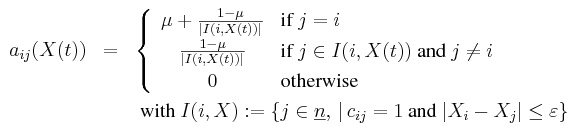(1)

The confidence matrix is nonnegative and each row sums up to one. The set I(i,X) is called the confidence set of agent i. The new opinion profile is then computed as: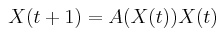(2)

2.9
The Parameter μ is not the same as the parameter μ in the DW model (Deffuant et al., 2001). To distinguish them we label the original parameter of the DW model μD. If we set μ = 1 - 2 μD and m = 2, then our model equals the DW model. The assumption of 0 < μD ≤ 0.5 that is made in DW model corresponds to 0 ≤ μ < 1 in our model.

2.10
All together we have presented a unified basic model of continuous opinion dynamics that allows for the analysis of the impact of different numbers of communicating agents. It includes a special class of communication regimes that is independent of social networks or, in other words, a communication regime that only has the fully connected network as its underlying social network. It incorporates two important basic models that are applied in many articles on continuous opinion dynamics with compromising agents, i.e. the model by Deffuant and Weisbuch and the model by Hegselmann and Krause. Actually both extremes happen in real social structures. But also meetings with m = 3 or 4 or 10 or 50 people occur. Independent variables of our model are the number of agents n, the number of communicating agents m, self-support μ, and the bounded confidence parameter ε. The initial profile X(0) and the randomly chosen m-communication regime matrices C(t) are also free variables of the model, but we will treat them as endogenous random choices being equally distributed within the defined bounds. Due to this randomness we are forced to run many simulations with different randomly chosen X(0) and C(t).

###Model analysis

3.1
In our model analysis we first show that the dynamics stabilize. Furthermore, we show that the dynamics are mean preserving for isolated fully connect groups of agents, and that the dynamics always reach a state where all agents are in isolated fully connected groups. An isolated fully connected group is a set of agents, in which all agents within the same group have a distance smaller than ε, while each agent outside the group has a distance larger than ε to each agent in the group. These results enable us to implement simulations that finally calculate the long-term limit of the dynamics.

#### Stabilizing and stable states

3.2
Proposition 3.1: Let X(0) ∈ Rn be an initial opinion profile, ε > 0 be a bound of confidence, μ ∈ [0,1] be a self-support, and m be the number of communication agents in each time-step. Furthermore, let C(t) be a random m-communication regime and X(t) be recursively defined as X(t) = A(X(t-1))X(t), the process of opinion dynamics. For this process, the following holds:
1. It reaches a stable state.

2. In finite time it reaches a state where all agents are in isolated fully connected groups.

3. An isolated fully connected group never splits.

4. The mean opinion of agents in an isolated fully connected group remains stable.
3.3
Proof: The stabilization theorem for continuous opinion dynamics (Lorenz, 2005) ensures the convergence of X(t) to a stable state when in each confidence matrix (i) the diagonal is positive, (ii) confidence is mutual (aij > 0 ⇔ aji>0), and (iii) there is a threshold δ such that the lowest positive entry is always greater than δ. All three conditions are fulfilled in every confidence matrix arising in the process: (i) Due to the fact that each agent is always closer than ε to himself and μ+ (1 - μ) / n > 0, (ii) because all agents have the same bound of confidence, and (iii) because every positive entry in a confidence matrix is always greater than 1-μ / n. This proves 1.

3.4
In a stable state each two agents must either have the same opinion or have a distance larger than ε. This is proved in Lorenz (2007, Section 3.3.5). It is trivial for the DW model and not totally trivial for the HK model. The proof extends naturally from the HK model to communication regimes with m < n. Thus, in the stable state agents are in isolated fully connected groups that have internal consensus. The distance between the two groups must be greater than ε, thus there is a time step t such that all agents are connected to each agent in his isolated fully connected group and has a distance of more than ε to all other agents. This proves 2.

3.5
The opinions in an isolated fully connected group can only take new opinions as average of the opinions in their isolated fully connected group; thus, the maximal distance of opinions in a fully connected group is by definition less than ε and cannot increase. This proves 3.

3.6
Consider that all agents in an opinion profile X(t) are in isolated fully connected groups. Then, regardless of the selection of the m communicating agents the confidence matrix A(X(t)) will be symmetric (not only with mutual confidence). It is easy to see that in this case the mean of all opinions remains stable.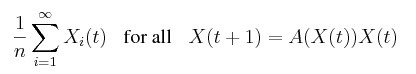(3)

As this holds for the means within all isolated fully connected groups, this proves 4.

3.7
Proposition 1 gives us a tool to optimize our simulations. First of all, if agents are in a state that is characterized by fully connected groups, then the average opinions in these groups do not change and thus, because fully connected groups do not split further, we can calculate the long-term limit which these groups independently converge to. Because the system reaches such a state in a finite time, we can implement our simulations as a process that simulates the opinion dynamics and - from time to time - checks if groups are fully connected. If groups are fully connected they can be collapsed such that all agents in this group have the same opinion given by the groups' average opinion. We stop simulation if all groups are collapsed. In fact, our results are equivalent to simulating the system for an infinitely long time.

#### Comparing limit states

3.8
For comparing final states of opinion dynamics, ideally, we would look at the distribution of clusters and agents within the opinion space. However, it is often useful and common practice to describe the final state by a one-dimensional value. Different measures are possible and different measures were used. For defining them let sSNC be the final number of clusters and let s1, s2 to s SNCbe the sizes of all clusters ordered by size such that i < j ↔ si ≥ sj. Let Oi be the opinion of cluster i. The different statistics are defined as follows:
• The number of clusters SNC is self-explaining. This measure is used by Urbig (2003). A weakness of this statistic is the fact that it ignores information by not refering to the cluster sizes. Thus, a single agent that is lost at the extreme counts as much as the remaining population of agents that perhaps clustered around a medium opinion. Such small minorities appear systematically in systems that we look at; Ben-Naim et al. (2003) show this especially for m = 2.

• The maximum cluster size SMC = s1 is a measure of the power of the majority and is used by Lorenz and Urbig (2007). It abstracts from minorities because only the largest majority counts.

• The Herfindahl index SHI is used in economic theory to describe the concentration of markets. It is based on cluster sizes but does not take into account the opinions that clusters are located at. Weisbuch et al. (2001) use it for describing limit states of opinion dynamics.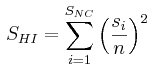(4)

• The Gini coefficient SGC is a measure of inequality and refers also to opinions of every single agent and thus takes cluster positions and indirectly the number of clusters into account. It is mainly used in the social sciences and welfare economics.(5)

• The number of major clusters SNMC that excludes minorities could be interesting as well. Deffuant et al. (2001) (in Figure 4) report such a statistic. However, they only exclude small extreme clusters at the ends of the opinion space but not all minorities, which probably did not appear that often. Based on our own simulation experience we will exclude all cluster that have a size below
½ · ε · n.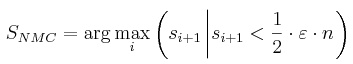(6)

###Simulations

4.1
Our simulations explore three basic questions. First, we run a typical example for opinion dynamics and compare the different descriptions of final states. Second, we investigate the effect that the number of individuals who met at one time, i.e. parameter m, has on the dynamics. Thereby we also look at different population sizes n. Finally we look at the effect of the self-support μ and how it may interact with m. An analysis of variance sheds light on the impact that these variables have.

#### Different descriptions of final states

4.2
For our first analysis we consider a population of 100 individuals and vary the bounded confidence parameter ε, while keeping the self support μ constant at zero. Figure 1 plots the different statistics on the final state for different values of ε and for the DW model (left, n = 100 and m = 2) and for the HK model (right, m = n = 100). The data points are adjusted such that they all start at the lower left corner that represents the case with the smallest chance for consensus and end in the upper right corner that represents the case with the largest chance for consensus. Due to these transformations, the comparisons of the absolute values of these curves do not have any meanings. However, we are able to compare the general shape of these curves.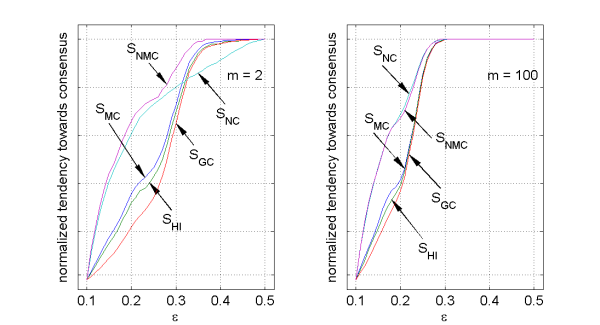Figure 1. Statistics on the final states for varying ε for m = 2 (left) and m = 100 (right) with n = 100 and μ = 0.0 (click to enlarge the figure)
4.3
We observe that Gini coefficient SGC, Herfindahl index SHI, and maximum cluster size SMC behave rather similar and consistently over the two values of m. Either they measure the same or what they measure is strongly correlated over these two settings. The maximum cluster size SMC is closely related to the Herfindahl index SHI. The latter includes more information, while the first ignores all clusters besides the largest cluster. We do not want to get into a longer discussion on the Gini coefficient, but we just want to mention that the Gini coefficient increases with larger clusters, which makes it closely related to the Herfindahl index. However, the Gini coefficient additionally incorporates information about the position of these clusters. The cluster number SNC and the number of majorities SNMC behave rather similar for the case m = n but differ in their shape for m = 2. In fact, while Ben-Naim et al. (2003) show that minorities (very small clusters) appear systematically for m = 2 (DW model) Lorenz (2007) shows that minorities do not systematically appear for the case m = n (HK model), except that a single central cluster might get relatively small. This occurrence of minorities is the reason why SNC and SNMC closely follow each other for m = n, but differ significantly for m = 2. This is also the reason why the Herfindahl index, Gini coefficient, and maximum cluster size seem to differ slightly more for m = 2 than for m = n; they simply treat minorities slightly different.

4.4
For the following analysis we focus on three statistics: SNC, SNMC, and SGC. We take the first two statistics because they measure something partially uncorrelated. The third is chosen from a group of three statistics that all seem to behave rather similar. To our knowledge, the Gini coefficient has not been explored as a statistics for final states in opinion dynamics; thus, we selected this statistic for our further analysis.

#### Changing the number of communicating agents

4.5
For exploring the effect of changing the number of communicating agents, m, we keep parameter μ constant at zero. For m = n the model reduces to the HK model and for m = 2 we get the DW model with μD = 0.5. The effect of changing parameter μ is explored later in this paper.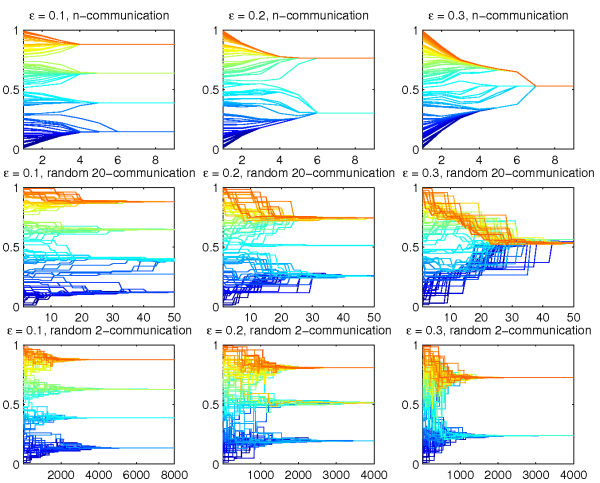Figure 2. Examples: 100 agents with randomly chosen initial opinions for ε from {0.1, 0.2, 0.3} and for m = n (HK model), m = 20 and m = 2 (DW model) (click to enlarge the figure)

4.6
We run nine simulation to get an initial idea of how m influences the dynamics. Figure 2 shows the nine processes of opinion dynamics, ε ∈ {0.1, 0.2, 0.3}, m ∈ {2, 20, 100}), and all with the same initial profile of 100 randomly chosen opinions. In each plot, the x-axis is time and the y-axis represents the opinion space. For low ε the agents form several clusters. For higher ε the number of clusters decreases. With even higher ε the agents find consensus. All models show the same behaviour but it seems that the same ε causes slightly more clusters in the DW
model.

4.7
To make the dynamics more visible let us consider a very simple example with three agents. Thereby we assume that for different numbers of agents the fundamental micro behaviours will not change significantly. Consider three agents with opinions 0, 0.5, and 1, all with ε = 0.5 and any self-support μ < 1, e.g. μ = 0.0. For the DW model two clusters will emerge, either with opinions 0.0 and 0.75 or with 0.25 and 1.0 (depending on the first communications). If the extreme agents communicate they ignore each other. If the middle agent communicates with an extreme agent it will adapt to the extreme and leave the space where the other extreme agent could influence the middle agent. For the HK model one cluster emerges with opinion 0.5. If we further increase ε above 0.5 then the probability to reach consensus increases for the DW model until finally the probability reaches 1. Between the extremes the particular patterns of communication influence the probability of convergence to one cluster.

4.8
All together we can state our first hypothesis: The higher the number of communicating agents, i.e. the larger m, the less the number of expected clusters.

4.9
This hypothesis is investigated by simulations, where the bounded confidence parameter ε and the number of agents who meet at once m change systematically. Figure 3 visualizes our simulation results using the number of clusters SNC, number of major clusters SNMC, and the Gini coefficient SGC. The Figure plots the results for n ∈ {100, 500, 1000}. For each data point we have at least 2.000 independent runs.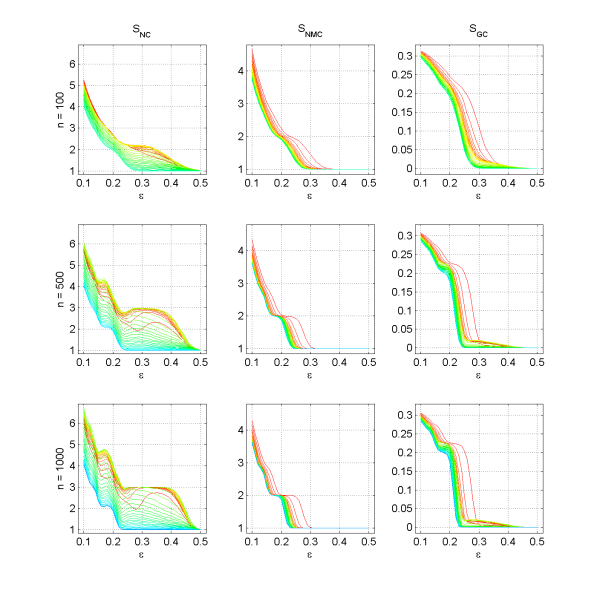Figure 3. Number of clusters SNC, number of major clusters SNMC, and Gini coefficient SGC for three populations sizes, n ∈ {100, 500, 1000} with μ = 0.0. The lines represent different levels of m (from blue over green and yellow to red, blue represents m = n and red represents m = 2). (click to enlarge the figure)

4.10
Let us first look at the number of major clusters SNMC. We observe that there is indeed a general tendency that a larger m increases the tendency towards consensus and thus towards less clusters. The effect remains stable also for increasing the population size.

4.11
If we look at the number of clusters SNC we observe two interesting effects. First, the effect is large and gets larger for an increasing number of agents. However, for small values of m the number of clusters increases for an increasing m while after a specific threshold it decreases again. Also interesting is the observation that the number of clusters is not monotonically decreasing in the confidence threshold ε, especially for large n. We attribute this effect to the occurrence of minorities. For the HK model the effect is rather small, because only one minority, i.e. the central cluster, may appear or disappear.

4.12
The Gini coefficient reflects the effects similarly, but with a much smaller emphasis on small clusters. While for small values of ε the Gini coefficient looks pretty much the same as the number of major clusters, for large values of ε one can also observe the effect that an increasing m first increases and finally decreases the Gini coefficient. Because this effect is almost invisible when looking at the number of major clusters, it is obviously an effect due to the presence of minorities. This observation also supports that the choice of the threshold for the size that determines a major clusters was somewhat reasonable.

4.13
We can summarize our simulation results by stating that meeting more agents at once decreases the number of major clusters and thus increases the tendency for consensus. The effect does not disappear for larger populations, but might get even more pronounced.

#### Changing the self-support

4.14
For analysing the effect of m we set μ to zero, because for this case both extremes, m = 2 and m = n, represent instances of the original DW model and HK model, respectively. If we change μ then for m = n we do not have the original HK model anymore, because the HK model is specified without a parameter describing how much agents adapt their opinions. We end up with a model that incorporates self-support into the HK model. We now analyse how changing this parameter μ affects the opinion dynamics.

4.15
Let us take a second look at the simple three-agent example introduced above. Consider the example for the DW model and ε bigger than 0.5. The slower the middle agent moves, i.e. the higher its self-support, the lower is the probability that it "loses contact" to one of the extreme agents. And the lower this probability the higher is the probability that the middle agent forces the extreme agents to reach consensus. Hence we can hypothesize that there are cases where a higher self-support decreases the expected number of clusters.

4.16
However, the slower the extreme agents move, the higher is the probability that the middle agent looses contact to an extreme agent, because the extreme agents do not get closer to the middle agent quickly enough. We thus might expect cases where an increase of the self-support parameter increases the number of evolving clusters. Hence, we can also hypothesize that there are cases where the number of clusters increases due to an increase in the self-support μ.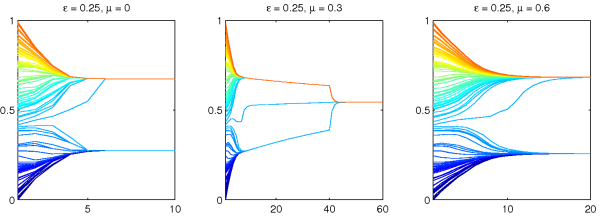Figure 4. Examples for one initial opinion profile X(0) ∈ R100, ε = 0.25 and μ = 0, 0.3, 0.6 (click to enlarge the figure)
4.17
To see that both effects are working see Figure 4, where we consider an example with a fixed initial opinion profile of 100 agents, m = 100 (remember that there is no randomness in the n-random communication regime, i.e. the HK model), ε = 0.25, and three stages for the parameter μ. We see that increasing μ from 0.0 to 0.3 takes the number of clusters from 2 to 1, but increasing μ further from 0.3 to 0.6 takes it back to 2 clusters. Many repeated simulations will show how these contrary forces work together in general (see figure 5).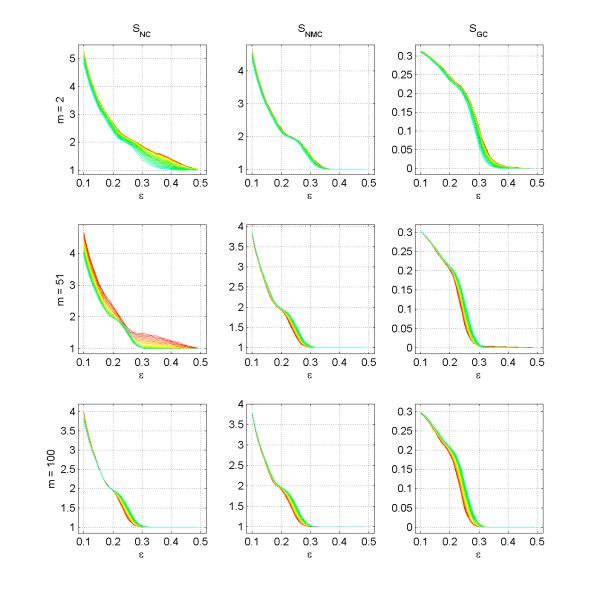Figure 5. Number of clusters SNC, number of major clusters SNMC, and Gini coefficient SGC for three different numbers of peers met at one m ∈ {2, 51, 100} with n = 100. The lines represent different levels of μ (from blue over green and yellow to red, blue represents μ = 0.9 and red represents μ = 0.0). (click to enlarge the figure)

4.18
Figure 5 illustrates how μ affects on average the final state of the opinions dynamics in the DW model (m = 2), in the HK model (m = n), and in an intermediate case (m = 51), respectively. We run simulations for 30 stages of ε and 30 stages for μ, while every setting was simulated at least 1.000 times with random initial opinion profiles.

#### Analysis of variance and ranking the factors

4.19
We can see that for the DW model (m = 2) an increase of μ decreases the number of clusters SNC. For the HK model (m = n = 100) we recognize a different case. For small ε the direction of the effect is the same as for the DW model (but the effect is much smaller); but for big ε it is inverse (but still the effect is smaller than for m = 2). In fact, an increase in μ for big ε increases the average number of clusters. The same interaction effect with m can be observed for the number of major clusters as well as for the Gini coefficient. However, the magnitude of these effects is smaller. The reason can be found in the existence of minorities in the DW model and the absence of them in the HK model. This is further supported by the fact that the two plots for the overall number of clusters SNC, which gives a large weight to minorities, for models with m < n, where minorities systematically appear, look structurally different from the other plots.

4.20
So far we have only looked at the averages of our simulation runs and have reasoned based on visual inspection of the plots. We did some sample calculations and have found that due to the large number of repetitions the differences between the extreme cases, i.e. m = 2 and m = n as well as between μ= 0.0 and μ= 0.9 are mostly statistically significant. However, we now have a more detailed look at the variances associated with different settings and we will look at how much variance can be attributed to variations in different parameters.
 Figure 6. Standard deviation of Number of clusters SNC, number of major clusters SNMC, and Gini coefficient SGC for three populations sizes, n ∈ {100, 500, 1000} where the lines represent different levels of m (blue represents m = n and red represents m = 2). This Figure complements Figure 3. (click to enlarge the figure)

 Figure 7. Standard deviation of Number of clusters SNC, number of major clusters SNMC, and Gini coefficient SGC for three different numbers of peers met at one m ∈ {2,51,100} with n = 100. The lines represent different levels of μ (from blue over green and yellow to red, blue represents μ = 0.9 and red represents μ = 0.0). This Figure complements Figure 5. (click to enlarge the figure)

4.21
We complement Figures 3 and 5 on the averages of our statistics with Figures 6 and 7 that plot the standard deviation for all these different settings. When ignoring minorities (SNMC) the pattern is very clear. Previous studies have shown that for specific values of ε opinion dynamics there are characteristic values that the dynamics stabilize at. Variance significantly increases for those parameters where the systems switches from one characteristic value to another one. The plots again support that for larger m these characteristic thresholds are smaller. Thus, for larger m less major clusters can be expected.

4.22
When we look at the case of the overall number of clusters SNC then we observe that the occurrence of minorities significantly destroys the clear pattern. First of all, we observe that for a larger self-support μ variance produced by the occurrence of minorities decreases. Furthermore, there is a more complex interaction between m and ε regarding the variance due to minorities.

4.23
In our previous analysis we have explained that some effects are smaller and some are larger. We now use an Analysis of variance (ANOVA) to quantify this a bit more precisely.5

4.24
Tables 1 and 2 present the results showing what proportion of the overall variation can be attributed to different factors and factor interactions. The within-groups (WG) variation reduces with larger n, which implies that the influence of the actual instantiation of the communication regime (besides m) and the initial opinion profile gets significantly smaller. The three parameters ε, m, and μ explain an increasingly large share of the variation, i.e. the between-groups (BG) variation. From both tables we can also conclude that the bounded confidence parameter ε is the most important driver, followed by the number of peers met at once m. The self-support μ is the least important driver. Looking at the proportions of variance attributed to changes in these parameters one can see that the differences are huge and that the ranking is therefore very clear. However, for the number of clusters including the minorities, m gets more and more important for larger n. This can be explained by the fact that with larger n, minorities appear more often for m = 2 but less often for m = n. The effect of ε decreases in n for the number of clusters but increases for major clusters and the Gini coefficient. The interaction effect between ε and m is small compared to the main effects but large compared to the effect of μ, and this interaction effect gets larger for larger values of n. Compared to the main effect of μ, the interaction effect of μ with m is comparatively large (similar size).

 Number of agents n Sum of squares Sources of variation in proportions of sum of squares WG BG ε m ε * m NC 100 3,151,083 0.2340 0.7660 0.6983 0.0535 0.0142 500 4,356,244 0.1806 0.8194 0.5993 0.1831 0.0370 1000 4,964,100 0.1475 0.8525 0.5785 0.2336 0.0404 NMC 100 1,862,348 0.1173 0.8827 0.8673 0.0074 0.0080 500 1,585,191 0.0860 0.9140 0.8983 0.0055 0.0102 1000 1,562,018 0.0726 0.9274 0.9098 0.0053 0.0124 GI 100 35,779 0.1325 0.8675 0.8485 0.0093 0.0097 500 32,460 0.0709 0.9291 0.9040 0.0089 0.0162 1000 31,585 0.0524 0.9476 0.9173 0.0093 0.0211
Table 1: Analysis of variance for μ = 0.0 and different n regarding ε and m

 Sum of squares Sources of variation in proportions of sum of squares WG BG ε m μ ε * m ε * μ m * μ ε * m * μ NC 3,362,997 0.1486 0.8514 0.7716 0.0521 0.0034 0.0193 0.0012 0.0029 0.0009 NMC 2,643,892 0.1171 0.8829 0.8482 0.0177 0.0001 0.0154 0.0005 0.0005 0.0005 GI 47.040 0.1433 0.8567 0.8133 0.0187 0.0001 0.0218 0.0011 0.0008 0.0009
Table 2: Analysis of variance for n=100 regarding ε, m, and μ

###Conclusion and outlook

5.1
This article has three contributions to the literature on opinion dynamics. First we introduce a model that unifies two frequently used models of opinion dynamics, namely the model by Deffuant and Weisbuch and the model by Hegselmann and Krause. Furthermore we have shown that the difference between these two models, i.e. the number of peers met at once, affects the tendency towards consensus. More precisely, the more agents are met at once, the less major clusters one can expect. However, for minor clusters this holds only for larger values of m. For smaller values of m the total number of clusters might increase with an increasing m. Additionally, we briefly discussed different statistics that could be used to describe final states of opinion dynamics. We used three of them to analyse the unifying model.

5.2
Regarding our analysis we can summarize our results. With larger population sizes the variance driven by initial opinions and random elements in the communication regime reduces, i.e. they get less and less important. With larger samples minorities get more and more important for m < n. On the number of major clusters, the bounded confidence parameter ε has the largest impact with distinction. Number of peers met at once m and self-support μ have a small impact while the latter has the smallest impact. Larger m decrease the number of major clusters.

###Acknowledgments

This article is a substantial extension of a work presented at the Second Conference of the European Social Simulation Association (ESSA), September 16-19, 2004, Valladolid, Spain. We want to thank participants of this conference as well as two anonymous reviewers, who gave critical comments that finally improved the paper very much. We want to thank Aljoscha Kaplan for comments that finally improved the language. All remaining errors remain ours.

###Notes

1We stress the matrix formalism because it is very general and an important proposition in the following part of the paper relies on it. Nevertheless, in this paper only random m-communication regimes are of interest.

2The term 'fully connected' here is not the same as strongly connected in network theory.

3The HK model is not mean-preserving. For instance, with ε = 0.3, μ = 0 and opinion vector (0.2, 0.5, 0.7) one get (0.35, 0.46, 0.6) after one step, which increases the mean from 0.46 to 0.472. Since the HK model is a specific case of our model, also our model is not mean-preserving.

4We have plotted the distribution of cluster sizes and there was a minimum between the peaks of minorities and the peak of majorities that varied approximately as our chosen threshold behaves.

5We are aware that given the previous plots on the standard deviation in different settings, a central assumption of ANOVA, i.e. the homogeneity of variances, is not fulfilled. Nevertheless, the results of the analysis reveal sufficiently huge differences, such that it still sheds some light on the model.

###References

AMBLARD, F., & DEFFUANT, G. (2004). The role of network topology on extremism propagation with the relative agreement opinion dynamics. http://arxiv.org/abs/cond-mat/0404574.

BEN-NAIN, E., KRAPIVSKY, P., VAZQUEZ, F., & REDNER, S. (2003). Unity and discord in opinion dynamics. Physica A, 330, 99–106.

DEFFUANT, G., AMBLARD, F., WEISBRUCH, G., & FAURE, T. (2002). How can extremism prevail? a study on the relative agreement interaction model. Journal of Artificial Societies and Social Simulation 5(4). <https://www.jasss.org/5/4/1.html>

DEFFUANT, G., SKERRATT, S., AMBLARD, F., FERRAND, N., CHATTOE, E., GILBERT, N., & WEISBRUCH, G. (2001). Improving Agri-environmental Policies: a Simulation Approach to the Cognitive Properties of Farmers and Institutions. Final Report, version 2, FAIR3 CT 2092 < http://wwwlisc.clermont.cemagref.fr/ImagesProject/freport.pdf >.

FORTUNATO, S. (2004, march). The Krause-Hegselmann consensus model with discrete opinions. arXiv:cond-mat/0403670v1.

HEGSELMANN, R., & KRAUSE, U. (2002). Opinion dynamics and bounded confidence models: Analysis, and simulation. Journal of Artifical Societies and Social Simulation, 5(3). <https://www.jasss.org/5/3/2.html>

HEGSELMANN, R., & KRAUSE, U. (2004). Opinion Dynamics Driven by Various Ways of Averaging. Computational Economics, 25(4), 381-405.

JAGER, W., & AMBLARD, F. (2004). Uniformity, bipolarization and pluriformity captured as generic stylized behavior with an agent-based simulation model of attitude change. Computational & Mathematical Organization Theory, 10, 295–303.

LORENZ, J. (2005). A stabilization theorem for continuous opinion dynamics. Physica A, 355(1), 217–223.

LORENZ, J. (2007). Repeated Averaging and Bounded Confidence Modeling, Analysis and Simulation of Continuous Opinion Dynamics. Ph.D. thesis at Universität Bremen. (http://nbn-resolving.de/urn:nbn:de:gbv:46-diss000106688)

LORENZ, J., & URBIG, D. (2007). About the power to enforce and prevent consensus by manipulating communication rules. Advances in Complex Systems, 10(2), 251-269.

MASON, W. A., CONREY, F. R., & SMITH, E. R. (2007, August). Situating social influence processes: Dynamic, multidirectional flows of influence within social networks. Personality and Social Psychology Review, 11(3), 279–300.

MOWEN, J. C., & MINOR, M. (1998). Consumer behavior. Prentice-Hall.

SCHWEITZER, F., & HOLYST, J. A. (2000). Modelling collective opinion formation by means of active brownian particles. The European Physical Journal B, 15, 723–732.

STAUFFER, D., SOUSA, A., & SCHULZE, C. (2004). Discretized opinion dynamics of the deffuant model on scale-free networks. Journal of Artificial Socienties and Social Simulation, 7(3).<https://www.jasss.org/7/3/7.html>

URBIG, D. (2003). Attitude dynamics with limited verbalisation capabilities. Journal of Artificial Societies and Social Simulation , 6(1). <https://www.jasss.org/6/1/2.html>

WEISBUCH, G., DEFFUANT, G., AMBLARD, F., & NADAL, J. P. (2001). Interacting agents and continuous opinion dynamics. http://arxiv.org/abs/cond-mat/0111494.

WEISBUCH, G., DEFFUANT, G., AMBLARD, F., & NADAL, J. P. (2002). Meet, discuss, and segregate! Complexity, 7(3).